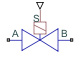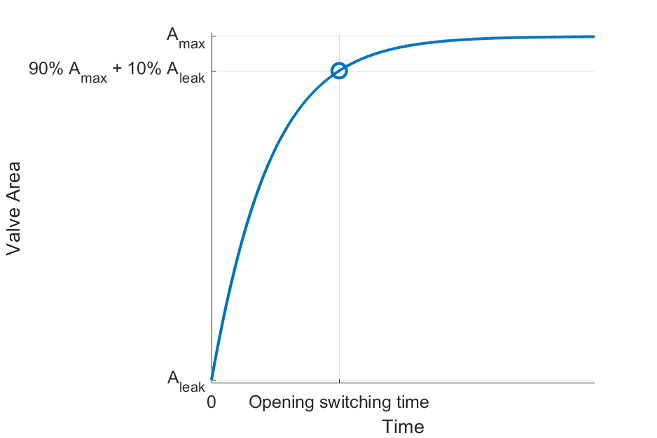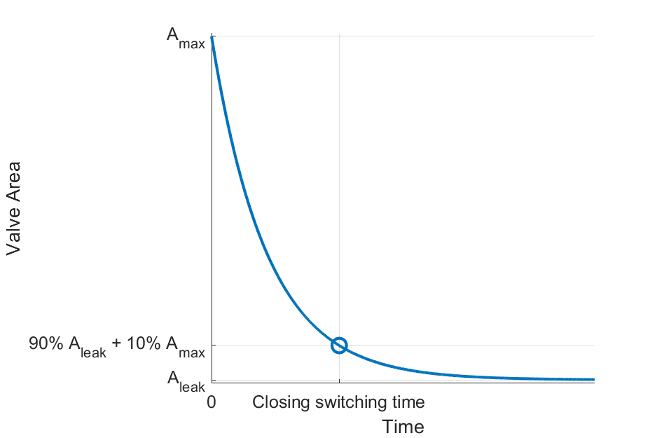# Solenoid Valve (IL)

Solenoid valve in an isothermal liquid system

Since R2023a

•Libraries:
Simscape / Fluids / Isothermal Liquid / Valves & Orifices / Directional Control Valves

## Description

The Solenoid Valve (IL) block models flow through a solenoid valve in an isothermal liquid network. The valve consists of a two-way directional control valve with a solenoid actuator. The physical signal at port S controls the solenoid. When the signal at port S is above 0.5, the solenoid turns on and the valve opens. When the signal at port S falls below 0.5, the solenoid turns off, closing the valve.

A solenoid valves consists of a valve body with a spring-loaded plunger that is operated by an electric solenoid. When the solenoid is on, the magnetic force lifts the spool, allowing fluid to flow. When the solenoid is off, the spring pushes the plunger back in place, stopping flow. The block does not model the mechanics of the solenoid explicitly, but characterizes the opening and closing of the valve using the opening and closing switching times.

### Opening Dynamics

The block assumes that the solenoid behaves as a resistor-inductor series circuit, represented as

`$V=iR+L\frac{\partial i}{\partial t},$`

where:

• V is the voltage across solenoid inductor.

• R is the resistance of the solenoid inductor.

• L is the inductance of the solenoid.

• i is the current through the solenoid inductor.

The solenoid generates a force proportional to the current squared, ${\text{F}}_{mag}\propto {i}^{2}.$

The expression for the cross-sectional area of the valve, A(t), depends on whether the valve is opening or closing. When the valve is opening,

`$\begin{array}{l}A\left(t\right)=\frac{{A}_{max}-{A}_{leak}}{7}\left({e}^{-2\frac{t-{t}_{0}}{{\tau }_{open}}}-8{e}^{\frac{t-{t}_{0}}{{\tau }_{open}}}\right)+{A}_{max}\\ {t}_{0}={t}_{start}+\mathrm{log}\left(4-\sqrt{16-7\frac{{A}_{max}-{A}_{0}}{{A}_{max}-{A}_{leak}}}\right){\tau }_{open}.\end{array}$`

When the valve is closing,

`$\begin{array}{l}\text{A(t)=}\left({A}_{max}-{A}_{leak}\right){e}^{-\frac{t-{t}_{0}}{{\tau }_{close}}}+{A}_{leak}\\ {t}_{0}={t}_{start}+\mathrm{log}\left(\frac{{A}_{0}-{A}_{leak}}{{A}_{max}-{A}_{leak}}\right){\tau }_{close},\end{array}$`

where:

• Amax is the value of the Maximum opening area parameter.

• Aleak is the value of the Leakage area parameter.

• tstart is the time that the solenoid turns on or off.

• A0 is the valve area at the time the solenoid turns on or off.

• ${\tau }_{open}=\frac{-{t}_{switc{h}_{open}}}{\mathrm{log}\left(4-\sqrt{15.3}\right)},$ where tswitchopen is the value of the Opening switching time parameter.

• ${\tau }_{close}=\frac{{t}_{switc{h}_{close}}}{\mathrm{log}\left(0.1\right)},$ where tswitchclose is the value of the Closing switching time parameter.

### Switching Time

The block characterizes the solenoid valve by using the valve opening and closing switching times specified by the Opening switching time and Closing switching time parameters, respectively. The Opening switching time parameter is the time from the solenoid being turned on to the flow rate rising to 90% of the way between its maximum and minimum values.The Closing switching time parameter is the time from the solenoid being turned off to the flow rate falling to 10% of the way between its maximum and minimum values.### Assumptions and Limitations

• The maximum force of the solenoid is the same as the force generated by the spring. The block does not include a hard stop.

• The damping inside the solenoid and the pressure flow forces are negligible.

• The spool is balanced.

## Ports

### Input

expand all

Physical signal that controls the valve. When the signal at port S is above 0.5, the solenoid turns on and the valve opens. When the signal at port S falls below 0.5 the solenoid turns off, and the valve closes.

### Conserving

expand all

Liquid entry or exit port.

Liquid entry or exit port.

## Parameters

expand all

Whether the initial position of the valve is open or closed.

Time from the solenoid turning on to the flow rate reaching 90% of the way between its maximum and minimum values.

Time from the solenoid turning off to the flow rate falling to 10% of the way between its maximum and minimum values.

Cross-sectional area of the valve in the fully open position.

Sum of all the gaps when the valve is in the fully closed position. The block saturates any valve area smaller than this value to the specified leakage area. The leakage area contributes to numerical stability by maintaining continuity in the flow.

Cross-sectional area at the entry and exit ports A and B. The block uses this area in the pressure-flow rate equation that determines the mass flow rate through the valve.

Correction factor that accounts for discharge losses in theoretical flows.

Upper Reynolds number limit for laminar flow through the valve.

Whether the block accounts for pressure increase when fluid flows from a region of smaller cross-sectional area to a region of larger cross-sectional area.

 Zhang, X., Lu, Y., Li, Y. Zhang, C., and Wang, R. “Numerical calculation and experimental study on response characteristics of pneumatic solenoid valves.” Measurement and control 22 (9-10) (2019): 1382-1393.

 Zhang, J., Liu, P., Fan, L., and Deng, Y. “Analysis on Dynamic Response Characteristics of High-Speed Solenoid Valve for Electronic control Fuel Injection System.” Mathematical Problems in Engineering (2020).